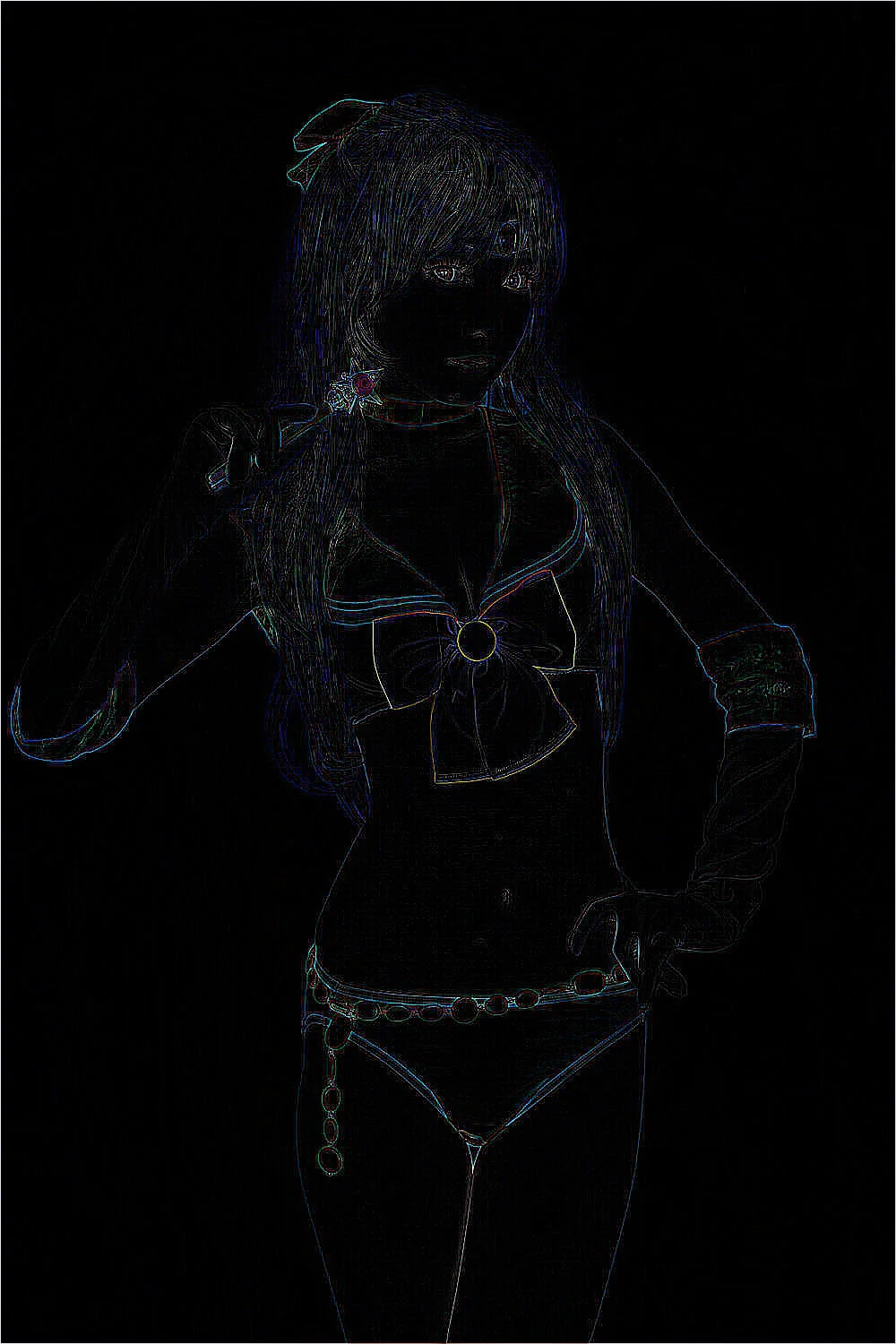# Python-PIL库的简单使用 图片的剪裁和批量添加水印鳄鱼君

## Image函数

#### Image.open(filename,mode)

```from PIL import  Image
img=Image.open('1.jpg')
#如果成功，open函数会返回一个图像对象JpegImageFile
print(type(img))
print(img.format,img.size,img.mode,img.getpixel((0,0)))
#结果:
#<class 'PIL.JpegImagePlugin.JpegImageFile'>
#JPEG (1400, 933) RGB
```

• format属性识别图形的源，若图片不是从文件读取的将显示None；
• size属性是一个包含宽度和高度的二元数组(以像素为单位)；
• mode属性定义了图像中波段的数量和名称，以及像素类型和深度，常见的模式是“L”(亮度)用于灰度图像，“RGB”用于真正的彩色图像，以及“CMYK”用于预压图像
• getpixel.获得某点像素用getpixel((w,h))可以直接返回这个点三个通道的像素值

#### Image.blend(image1,image2, alpha)

```from PIL import Image
img1=Image.open('1.jpg')
print(img1.size)
img2=Image.open('3.jpg')
print(img2.size)

new=Image.blend(img1,img2,0.3)
new.show()
```

#### Image.new(mode, size, color)

```from PIL import Image
img= Image.open("1.jpg")
new_img=Image.new('RGB', img.size, '#ff0000')
new_img.show()
```

```from PIL import Image
img1=Image.open('1.jpg')
img2=Image.open('2.png')

r,g,b=img1.split()
new=Image.composite(img1,img2,r)
new.show()
```

#### save(filename,format)

```from PIL import  Image
img=Image.open('1.jpg')
img.save('1.jpg','png')
print(img.format)
```

#### show()

```from PIL import  Image
img=Image.open('1.jpg')
img.show()
```

```from PIL import Image
import matplotlib.pyplot as plt
img=Image.open('1.jpg')
plt.figure('1')
plt.figure(num=1,figsize=(8,5),)
plt.title('the image title')#图像加标题
plt.axis('off')#不显示坐标轴
plt.imshow(img)
plt.show()
```
` `

figure默认是带axis的，如果没有需要，我们可以关掉。

#### matplotlib标准模式

```plt.figure(num=5, figsize=(8,5),)
#plt.figure(num='newimage', figsize=(8,5),)
plt.title('The image title', color='#0000FF')
plt.imshow(lena) # 显示图片
plt.axis('off') # 不显示坐标轴
plt.show()
```

## 常见图像的处理

#### thumbnail(size,resample)

```from PIL import  Image
img=Image.open('1.jpg')
size=(1000,1000)
img.thumbnail(size) #大小是元组长和宽
#img.save('2.jpg','JPEG')  以JPEG格式保存，结果就是jpg后缀
img.save('2.png','PNG') #以PNG格式保存，结果就是png后缀

```

#### crop(box)

```from PIL import  Image
img=Image.open('1.jpg')
box=(500,500,1000,1000)
reg=img.crop(box)
reg.show()
```

box是一个有四个数字的元组(upper_left_x,upper_left_y,lower_right_x,lower_right_y),分别表示裁剪矩形区域的左上角x,y坐标,右下角的x,y坐标,规定图像的最左上角的坐标为原点(0,0),宽度的方向为x轴，高度的方向为y轴，每一个像素代表一个坐标单位。crop()返回的仍然原Image对象。

box = (left, top, left+width, top+height)，所以你不能设置为(100,100,100,100)，呢样会报错SystemError: tile cannot extend outside image

#### transpose(method)

```from PIL import  Image
img=Image.open('1.jpg')
img_rotate_180=img.transpose(Image.FLIP_TOP_BOTTOM)
img_rotate_180.show()
```

method是transpose的参数，表示选择什么样的翻转或者旋转方式，可以选择的值有:

• Image.FLIP_LEFT_RIGHT,表示将图像左右翻转
• Image.FLIP_TOP_BOTTOM,表示将图像上下翻转
• Image.ROTATE_90,表示将图像逆时针旋转90°
• Image.ROTATE_180,表示将图像逆时针旋转180°
• Image.ROTATE_270,表示将图像逆时针旋转270°
• Image.TRANSPOSE,表示将图像进行转置(相当于顺时针旋转90°)
• Image.TRANSVERSE,表示将图像进行转置,再水平翻转（这点存在疑问```from PIL import  Image
img=Image.open('2.png')
img.save('2.jpg','png')#把png格式转换为jpg

img=Image.open('1.jpg')
reg=Image.open('2.jpg')
img.paste(reg,(10,10),None)#左上角
img.show()

```

region是要粘贴的Image对象,box是要粘贴的位置，可以是一个两个元素的元组，表示粘贴区域的左上角坐标,也可以是一个四个元素的元组，表示左上角和右下角的坐标。如果是四个元素元组的话,box的size必须要和region的size保持一致，否则将会被convert成和region一样的size。

#### split()

split()方法可以原来图像的各个通道分离,比如对于RGB图像，可以将其R,G,B三个颜色通道分离。 代码参考:

```from PIL import  Image
img=Image.open('1.jpg')
r,g,b=img.split()
r.show()
g.show()
b.show()
```#### merge(mode,channels)

merge方法和split方法是相对的，其将多个单一通道的序列合并起来，组成一个多通道的图像，mode是合并之后图像的模式，比如”RGB”,channels是多个单一通道组成的序列。

```from PIL import  Image
img=Image.open('1.jpg')
r,g,b=img.split()
img_mer=Image.merge('RGB',[r,g,b])
img_mer.show()
```

#### resize(size,resample,box)

resize方法可以将原始的图像转换大小,size是转换之后的大小,resample是重新采样使用的方法，仍然有Image.BICUBIC，PIL.Image.LANCZOS，PIL.Image.BILINEAR，PIL.Image.NEAREST这四种采样方法，默认是PIL.Image.NEAREST,box是指定的要resize的图像区域，是一个用四个元组指定的区域(含义和上面所述box一致)。具体的参数数值需要自己调试。

```from PIL import  Image
img=Image.open('1.jpg')
img_size=img.resize((500,500))
print(img_size)#<PIL.Image.Image image mode=RGB size=500x500 at 0x2561C18>

img_size.show()
img_size_box=img.resize((500,500),box=(0,0,250,250))
img_size_box.show()
```

#### convert(mode,matrix,dither,palette,colors)(mode转换)

convert方法可以改变图像的mode,一般是在’RGB'(真彩图)、’L'(灰度图)、’CMYK'(压缩图)之间转换。我们可以首先将图像转化为灰度图，再从灰度图转化为真彩图。值得注意的是,从灰度图转换为真彩图，虽然理论上确实转换成功了，但是实际上是很难恢复成原来的真彩模式的(不唯一)。

```from PIL import  Image
img=Image.open('1.jpg')
img_L=img.convert('L')
img_L.show()
print(img_L.mode)
img_rgb=img_L.convert('RGB')
img_rgb.show()
print(img_rgb.mode)
```

#### filter(filter)(应用过滤器)

filter方法可以将一些过滤器操作应用于原始图像，比如模糊操作，查找边、角点操作等。filter是过滤器函数，在PIL.ImageFilter函数中定义了大量内置的filter函数，比如BLUR(模糊操作),GaussianBlur(高斯模糊),MedianFilter(中值过滤器)，FIND_EDGES(查找边)等。

```from PIL import  ImageFilter
from PIL import  Image
img=Image.open('1.jpg')
img_blur=img.filter(ImageFilter.BLUR)
img_blur.show()
img_edg=img.filter(ImageFilter.FIND_EDGES)
img_edg.show()
img_gau=img.filter(ImageFilter.GaussianBlur)
img_gau.show()
img_med=img.filter(ImageFilter.MedianFilter)
img_med.show()

```#### point(lut,mode)(对图像像素操作)

point方法可以对图像进行单个像素的操作，上面的代码对point方法传入了一个匿名函数,表示将图像的每个像素点大小都乘以1.5,mode是返回的图像的模式，默认是和原来图像的mode是一样的。代码参考:

```from PIL import  Image
img=Image.open('1.jpg')
img_point=img.point(lambda x:x*1.5)
img_point.show()
img_point.save('2.jpg')
```#### ImageEnhance()(图像增强)

ImageEnhance是PIL下的一个子类，主要用于图像增强，比如增加亮度(Brightness),增加对比度(Contrast)等。上面的代码将原来图像的亮度增加50%,将对比度也增加了50%。

```from PIL import ImageEnhance
from PIL import Image
img=Image.open('1.jpg')
bright=ImageEnhance.Brightness(img)
img_bright=bright.enhance(1.5)
img_bright.show()
contrast=ImageEnhance.Contrast(img)
img_contrast=contrast.enhance(1.5)
img_contrast.show()

```#### ImageSequence()(处理图像序列)

```from PIL import ImageSequence
from PIL import Image
gif = Image.open("1.gif")
for i,frame in enumerate(ImageSequence.Iterator(gif),1):
if frame.mode == 'JPEG':
frame.save("%d.jpg" %i)
else:
frame.save("%d.png" % i)
```

```index = 0
while 1:
try:
gif.seek(index)
gif.save("%d.%s" %(index,'jpg' if gif.mode == 'JPEG' else 'png'))
index += 1
except EOFError:
print("Reach the end of gif sequence!")
break
```

#### ImageDraw图像添加文字

```from PIL import ImageDraw

from PIL import Image
from PIL import ImageFont
img=Image.open('1.jpg')
draw=ImageDraw.Draw(img)
width,height=img.size
print(width,height)

#选择文字字体和大小
font=ImageFont.truetype('font.ttf',200)#引入字体文件
#设置文字颜色
color='#ff0000'
#写入文字
draw.text((40,height-100),'E1yu.com',font=font,fill=color)
img.show()

```

## 文字水印的添加

```from PIL import Image
from PIL import ImageDraw
from PIL import ImageFont
img=Image.open('1.jpg')
draw=ImageDraw.Draw(img)
color='#ff0000'
draw.text((0,0),u'E1yu.com',font=font,fill=color)
img.show()
```

## 图片添加图片水印

```from PIL import Image
img=Image.open('1.jpg')
img_mark=Image.open('2.png')
img.paste(img_mark,(0,0))
img.show()
```

```from PIL import Image
im = Image.open("1.jpg")
mark=Image.open("2.png")
layer=Image.new('RGBA', im.size, (0,0,0,0))
layer.paste(mark, (0,0))
out=Image.composite(layer,im,layer)
out.show()
```

```import os
filename='G:/0a图库'
img_list=os.listdir(filename)#listdir会返回当前文件夹下的所有文件
for img in img_list:
print(img)
```

```from PIL import Image

import os
filename='G:/0a图库'#存储图片的目录文件
img_list=os.listdir(filename)
img_mark=Image.open('2.png')
for img in img_list:#循环遍历所有图片的文件名
new_img=Image.open(filename+'/'+img)
layer=Image.new('RGBA',new_img.size)#新建一个图层，颜色默认就是透明
layer.paste(img_mark,(0,0))
out=Image.composite(layer,new_img,layer)
out.show()
```

```from PIL import Image
import os
#获取文件夹下的所有图像文件
def get_img(filepath,water_mark,save_path):
try:
#判断当前文件夹下所有文件的格式,不需要可删除
img_fillter=['png','jpg']
for img in os.listdir(filepath):
if img.split('.')[-1] in img_fillter:
img_path=filepath+'/'+img
img_watermark(img_path,water_mark,save_path)
except Exception as e:
print(e)
#图片添加水印
def img_watermark(img_path,water_mark,save_path):
try:
img=Image.open(img_path) #打开图片
img_mark=Image.open(water_mark)#打开水印图片
layer=Image.new('RGBA',img.size)
layer.paste(img_mark,(img.size-img_mark.size,img.size-img_mark.size))#水印在图片右下角,(0,0)左上角
out=Image.composite(layer,img,layer)
out.save(save_path+'/new_'+img_path.split('/')[-1])
except Exception as e:
print(e)
get_img('G:/0a图库','2.png','G:/0b图库')
```

### 文章对你有帮助可赏作者一杯咖啡

#### 支付宝扫一扫打赏#### 微信扫一扫打赏Vieu4.6主题Q Q 登 录